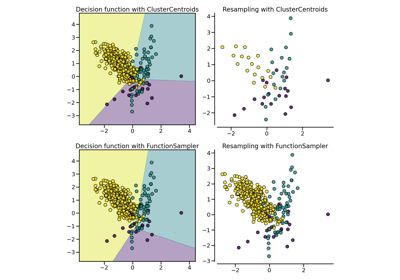# `imblearn.under_sampling`.AllKNN¶

class `imblearn.under_sampling.``AllKNN`(sampling_strategy='auto', n_neighbors=3, kind_sel='all', allow_minority=False, n_jobs=None)[source]

Undersample based on the AllKNN method.

This method will apply ENN several time and will vary the number of nearest neighbours.

Read more in the User Guide.

Parameters
sampling_strategystr, list or callable

Sampling information to sample the data set.

• When `str`, specify the class targeted by the resampling. Note the the number of samples will not be equal in each. Possible choices are:

`'majority'`: resample only the majority class;

`'not minority'`: resample all classes but the minority class;

`'not majority'`: resample all classes but the majority class;

`'all'`: resample all classes;

`'auto'`: equivalent to `'not minority'`.

• When `list`, the list contains the classes targeted by the resampling.

• When callable, function taking `y` and returns a `dict`. The keys correspond to the targeted classes. The values correspond to the desired number of samples for each class.

n_neighborsint or object, default=3

If `int`, size of the neighbourhood to consider to compute the nearest neighbors. If object, an estimator that inherits from `sklearn.neighbors.base.KNeighborsMixin` that will be used to find the nearest-neighbors.

kind_sel{‘all’, ‘mode’}, default=’all’

Strategy to use in order to exclude samples.

• If `'all'`, all neighbours will have to agree with the samples of interest to not be excluded.

• If `'mode'`, the majority vote of the neighbours will be used in order to exclude a sample.

allow_minoritybool, default=False

If `True`, it allows the majority classes to become the minority class without early stopping.

New in version 0.3.

n_jobsint, default=None

Number of CPU cores used during the cross-validation loop. `None` means 1 unless in a `joblib.parallel_backend` context. `-1` means using all processors. See Glossary for more details.

`CondensedNearestNeighbour`

Under-sampling by condensing samples.

`EditedNearestNeighbours`

Under-sampling by editing samples.

`RepeatedEditedNearestNeighbours`

Under-sampling by repeating ENN.

Notes

The method is based on [Rc251c8440626-1].

Supports multi-class resampling. A one-vs.-rest scheme is used when sampling a class as proposed in [Rc251c8440626-1].

References

Rc251c8440626-1(1,2)

I. Tomek, “An Experiment with the Edited Nearest-Neighbor Rule,” IEEE Transactions on Systems, Man, and Cybernetics, vol. 6(6), pp. 448-452, June 1976.

Examples

```>>> from collections import Counter
>>> from sklearn.datasets import make_classification
>>> from imblearn.under_sampling import AllKNN
>>> X, y = make_classification(n_classes=2, class_sep=2,
... weights=[0.1, 0.9], n_informative=3, n_redundant=1, flip_y=0,
... n_features=20, n_clusters_per_class=1, n_samples=1000, random_state=10)
>>> print('Original dataset shape %s' % Counter(y))
Original dataset shape Counter({1: 900, 0: 100})
>>> allknn = AllKNN()
>>> X_res, y_res = allknn.fit_resample(X, y)
>>> print('Resampled dataset shape %s' % Counter(y_res))
Resampled dataset shape Counter({1: 887, 0: 100})
```
Attributes
sample_indices_ndarray of shape (n_new_samples)

Indices of the samples selected.

New in version 0.4.

`__init__`(self, sampling_strategy='auto', n_neighbors=3, kind_sel='all', allow_minority=False, n_jobs=None)[source]

Initialize self. See help(type(self)) for accurate signature.

`fit`(self, X, y)[source]

Check inputs and statistics of the sampler.

You should use `fit_resample` in all cases.

Parameters
X{array-like, dataframe, sparse matrix} of shape (n_samples, n_features)

Data array.

yarray-like of shape (n_samples,)

Target array.

Returns
selfobject

Return the instance itself.

`fit_resample`(self, X, y)[source]

Resample the dataset.

Parameters
X{array-like, dataframe, sparse matrix} of shape (n_samples, n_features)

Matrix containing the data which have to be sampled.

yarray-like of shape (n_samples,)

Corresponding label for each sample in X.

Returns
X_resampled{array-like, dataframe, sparse matrix} of shape (n_samples_new, n_features)

The array containing the resampled data.

y_resampledarray-like of shape (n_samples_new,)

The corresponding label of X_resampled.

`fit_sample`(self, X, y)[source]

Resample the dataset.

Parameters
X{array-like, dataframe, sparse matrix} of shape (n_samples, n_features)

Matrix containing the data which have to be sampled.

yarray-like of shape (n_samples,)

Corresponding label for each sample in X.

Returns
X_resampled{array-like, dataframe, sparse matrix} of shape (n_samples_new, n_features)

The array containing the resampled data.

y_resampledarray-like of shape (n_samples_new,)

The corresponding label of X_resampled.

`get_params`(self, deep=True)[source]

Get parameters for this estimator.

Parameters
deepbool, default=True

If True, will return the parameters for this estimator and contained subobjects that are estimators.

Returns
paramsmapping of string to any

Parameter names mapped to their values.

`set_params`(self, **params)[source]

Set the parameters of this estimator.

The method works on simple estimators as well as on nested objects (such as pipelines). The latter have parameters of the form `<component>__<parameter>` so that it’s possible to update each component of a nested object.

Parameters
**paramsdict

Estimator parameters.

Returns
selfobject

Estimator instance.

## Examples using `imblearn.under_sampling.AllKNN`¶